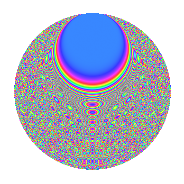# Properties

 Label 2304.2.bjLevel $2304$ Weight $2$ Character orbit 2304.bj Rep. character $\chi_{2304}(95,\cdot)$ Character field $\Q(\zeta_{24})$ Dimension $736$ Sturm bound $768$

# Related objects

## Defining parameters

 Level: $$N$$ $$=$$ $$2304 = 2^{8} \cdot 3^{2}$$ Weight: $$k$$ $$=$$ $$2$$ Character orbit: $$[\chi]$$ $$=$$ 2304.bj (of order $$24$$ and degree $$8$$) Character conductor: $$\operatorname{cond}(\chi)$$ $$=$$ $$288$$ Character field: $$\Q(\zeta_{24})$$ Sturm bound: $$768$$

## Dimensions

The following table gives the dimensions of various subspaces of $$M_{2}(2304, [\chi])$$.

Total New Old
Modular forms 3200 800 2400
Cusp forms 2944 736 2208
Eisenstein series 256 64 192

## Trace form

 $$736q + 24q^{5} - 16q^{9} + O(q^{10})$$ $$736q + 24q^{5} - 16q^{9} + 8q^{13} + 16q^{21} - 8q^{25} + 24q^{29} - 32q^{33} + 32q^{37} - 24q^{41} + 16q^{45} - 16q^{57} + 8q^{61} - 48q^{65} + 16q^{69} - 32q^{73} + 24q^{77} + 48q^{85} + 40q^{93} - 16q^{97} + O(q^{100})$$

## Decomposition of $$S_{2}^{\mathrm{new}}(2304, [\chi])$$ into newform subspaces

The newforms in this space have not yet been added to the LMFDB.

## Decomposition of $$S_{2}^{\mathrm{old}}(2304, [\chi])$$ into lower level spaces

$$S_{2}^{\mathrm{old}}(2304, [\chi]) \cong$$ $$S_{2}^{\mathrm{new}}(288, [\chi])$$$$^{\oplus 4}$$$$\oplus$$$$S_{2}^{\mathrm{new}}(1152, [\chi])$$$$^{\oplus 2}$$

## Hecke characteristic polynomials

There are no characteristic polynomials of Hecke operators in the database